# 训练堆叠自编码器进行图像分类

### 数据集

```% Load the training data into memory [xTrainImages,tTrain] = digitTrainCellArrayData; % Display some of the training images clf for i = 1:20 subplot(4,5,i); imshow(xTrainImages{i}); end```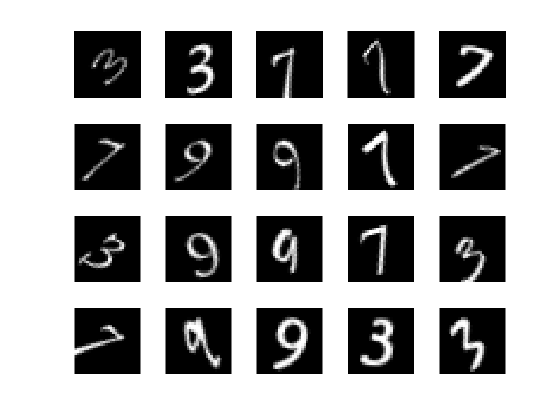### 训练第一个自编码器

`rng('default')`

`hiddenSize1 = 100;`

• `L2WeightRegularization` 控制 L2 正则项对网络权重（而不是偏置）的影响。这通常应该非常小。

• `SparsityRegularization` 控制稀疏正则项的影响，该正则项会尝试对隐含层的输出的稀疏性施加约束。请注意，这与将稀疏正则项应用于权重不同。

• `SparsityProportion` 是稀疏正则项的参数。它控制隐含层的输出的稀疏性。较低的 `SparsityProportion` 值通常导致只为少数训练样本提供高输出，从而使隐藏层中的每个神经元“专门化”。例如，如果 `SparsityProportion` 设置为 0.1，这相当于说隐藏层中的每个神经元针对训练样本的平均输出值应该为 0.1。此值必须介于 0 和 1 之间。理想值因问题的性质而异。

```autoenc1 = trainAutoencoder(xTrainImages,hiddenSize1, ... 'MaxEpochs',400, ... 'L2WeightRegularization',0.004, ... 'SparsityRegularization',4, ... 'SparsityProportion',0.15, ... 'ScaleData', false);```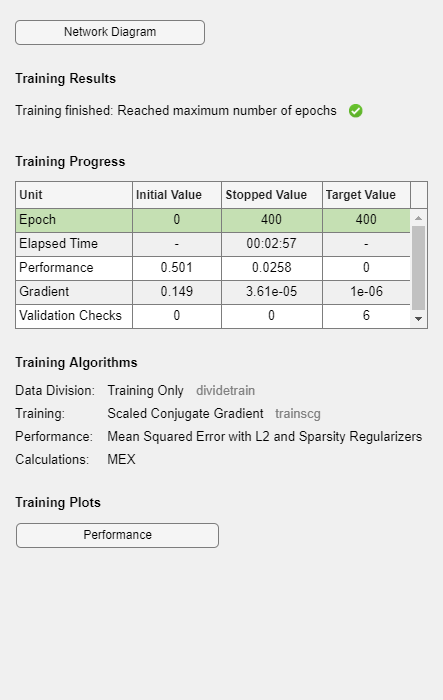`view(autoenc1)`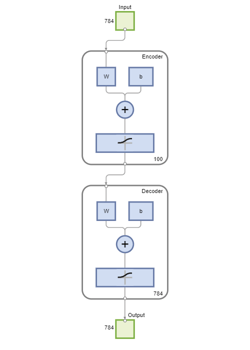### 可视化第一个自编码器的权重

```figure() plotWeights(autoenc1);```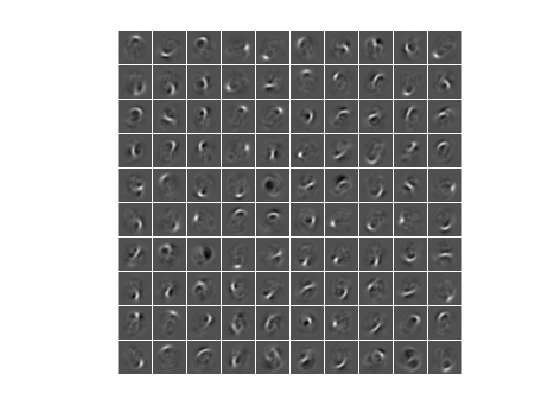`feat1 = encode(autoenc1,xTrainImages);`

### 训练第二个自编码器

```hiddenSize2 = 50; autoenc2 = trainAutoencoder(feat1,hiddenSize2, ... 'MaxEpochs',100, ... 'L2WeightRegularization',0.002, ... 'SparsityRegularization',4, ... 'SparsityProportion',0.1, ... 'ScaleData', false);```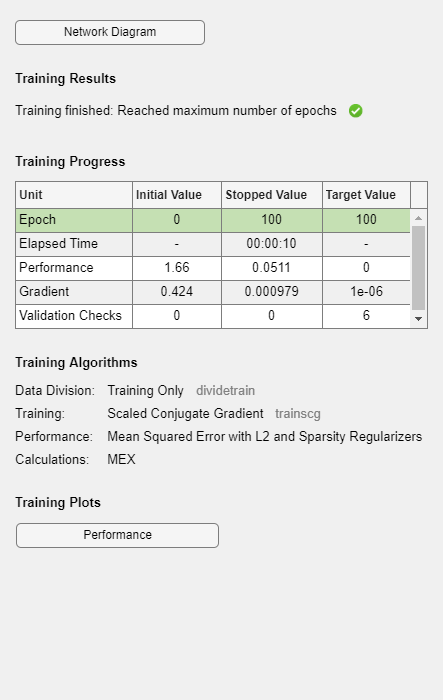`view(autoenc2)`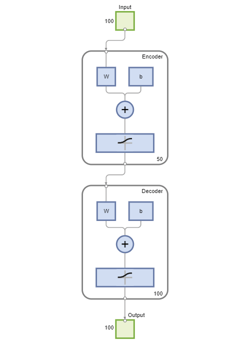`feat2 = encode(autoenc2,feat1);`

### 训练最终 softmax 层

`softnet = trainSoftmaxLayer(feat2,tTrain,'MaxEpochs',400);`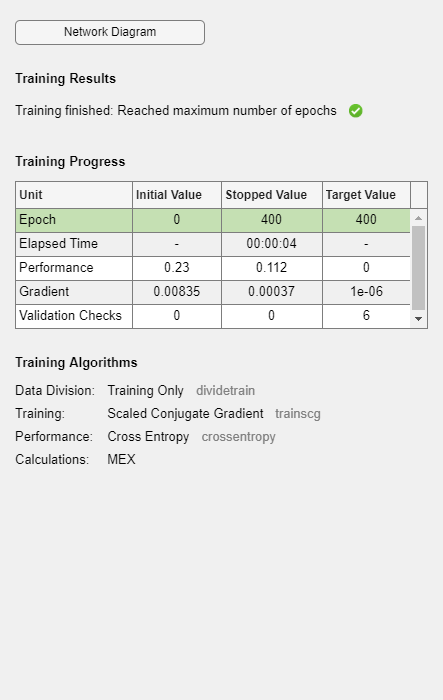`view(softnet)`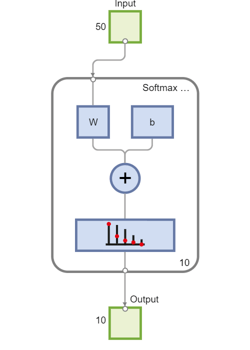### 形成堆叠神经网络

`view(autoenc1)``view(autoenc2)``view(softnet)``stackednet = stack(autoenc1,autoenc2,softnet);`

`view(stackednet)`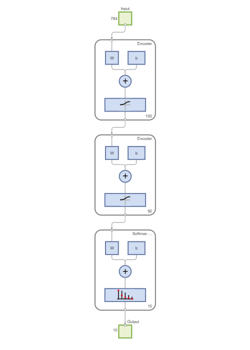```% Get the number of pixels in each image imageWidth = 28; imageHeight = 28; inputSize = imageWidth*imageHeight; % Load the test images [xTestImages,tTest] = digitTestCellArrayData; % Turn the test images into vectors and put them in a matrix xTest = zeros(inputSize,numel(xTestImages)); for i = 1:numel(xTestImages) xTest(:,i) = xTestImages{i}(:); end```

```y = stackednet(xTest); plotconfusion(tTest,y);```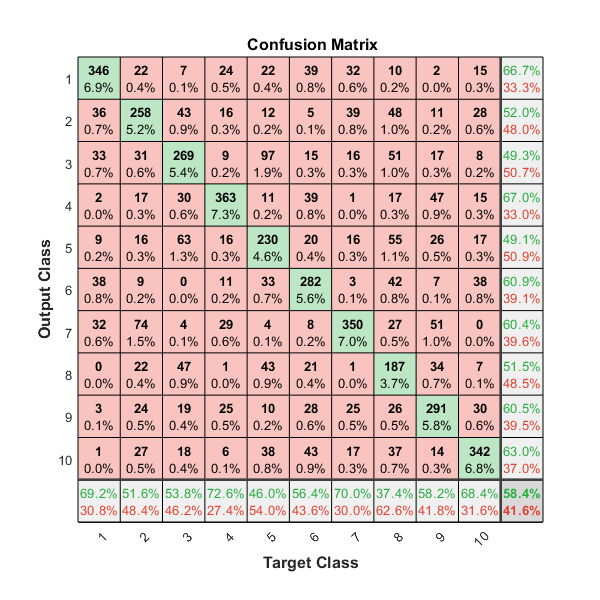### 微调堆叠神经网络

```% Turn the training images into vectors and put them in a matrix xTrain = zeros(inputSize,numel(xTrainImages)); for i = 1:numel(xTrainImages) xTrain(:,i) = xTrainImages{i}(:); end % Perform fine tuning stackednet = train(stackednet,xTrain,tTrain);```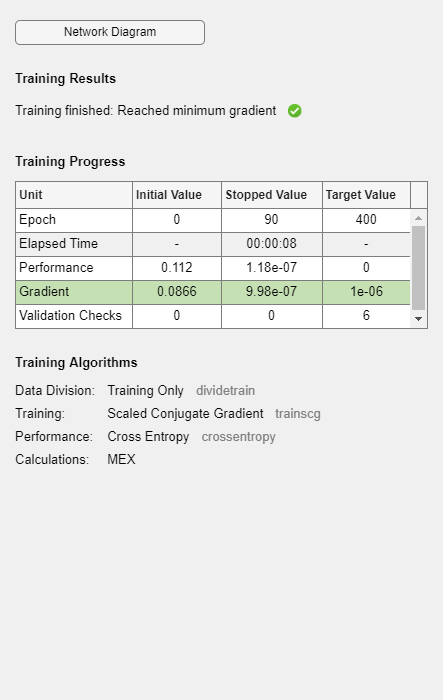```y = stackednet(xTest); plotconfusion(tTest,y);```